Interview Questions and Answers for 'Concerto software' | Search Interview Question - javasearch.buggybread.com# Search Interview QuestionsMore than 3000 questions in repository.There are more than 900 unanswered questions.Have a video suggestion.
Click Correct / Improve and please let us know.
Label / Company      Label / Company / TextSubmit Question

Interview Questions and Answers for 'Concerto software' - 3 question(s) found - Order By Newest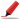Very frequently asked. Among first few questions in almost all interviews. Among Top 5 frequently asked questions. Frequently asked in Indian service companies (HCL,TCS,Infosys,Capgemini etc based on multiple feedback ) and Epam Systems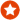Q1. Difference between == and .equals() ? Core Java
Ans. "equals" is the method of object class which is supposed to be overridden to check object equality, whereas "==" operator evaluate to see if the object handlers on the left and right are pointing to the same object in memory.

x.equals(y) means the references x and y are holding objects that are equal. x==y means that the references x and y have same object.

Sample code:

String x = new String("str");
String y = new String("str");

System.out.println(x == y); // prints false
System.out.println(x.equals(y)); // prints true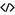Sample Code for equalsHelp us improve. Please let us know the company, where you were asked this question :LikeDiscussCorrect / Improvejava   string comparison   string   object class   ==    equals   object equality  operator   == vs equals   equals vs ==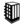Asked in 294 Companies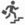basic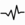frequent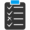Try 6 Question(s) TestRelated Questions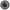What is the difference between = and == in Java ?How is == operator different for objects and primitive types ?What will be the output of following code

Integer x = 1;
Integer y = 2;
System.out.println(x == y);

What if you change 1 to "1" and Integer to String?Q2. What are the core OOPs concepts ? Core Java
Ans. Abstraction, Encapsulation, Polymorphism , Composition and InheritanceHelp us improve. Please let us know the company, where you were asked this question :LikeDiscussCorrect / Improvecore oops conceptsAsked in 65 CompaniesbasicfrequentRelated QuestionsWhat are the difference between composition and inheritance in Java?Explain OOPs

or

Explain OOPs Principles

or

Explain OOPs Concepts

or

Explain OOPs features

orWhat is the difference between Encapsulation and Abstraction?What are points to consider in terms of access modifier when we are overriding any method?What is Polymorphism in Java ?Difference between Overloading and Overriding ?Can you give a real world example of Encapsulation and Abstraction ?Why every object constructor automatically call super() in Object before its own constructors?How compiler handles the exceptions in overriding ?Difference between Composition and Inheritance ?Q3. Pascal Triangle Program
Ans. package snippet;

import java.util.ArrayList;
import java.util.Arrays;
import java.util.HashMap;
import java.util.List;
import java.util.Map;

public class Pascal {
public static void main(String[] args) {

System.out.println(generatePascalTriangle(5));

}

static Map> generatePascalTriangle(int size) {
Map> triangle = new HashMap<>();

triangle.put(0, Arrays.asList(1));
triangle.put(1, Arrays.asList(1, 1));

for (int i = 2; i <= size; i ) {
List coeffListForI = new ArrayList<>();
List coeffListForI_1 = triangle.get(i - 1);
for (int j = 0; j <= coeffListForI_1.size() - 2; j ) {
}
triangle.put(i, coeffListForI);
}

return triangle;
}
}Help us improve. Please let us know the company, where you were asked this question :LikeDiscussCorrect / ImproveAsked in 8 CompaniesRelated QuestionsDifference between == and .equals() ?Why is String immutable in Java ?Explain the scenerios to choose between String , StringBuilder and StringBuffer ?

or

What is the difference between String , StringBuilder and StringBuffer ?What are the difference between composition and inheritance in Java?Explain OOPs

or

Explain OOPs Principles

or

Explain OOPs Concepts

or

Explain OOPs features

orWhat is a Lambda Expression ? What's its use ?Does garbage collection guarantee that a program will not run out of memory?Why Char array is preferred over String for storing password?What are different ways to create String Object? Explain.What is the difference between final, finally and finalize() ?